# Single subject analysis¶

This section covers useful analysis techniques that can be used to estimate linear regression effects on a single subject level.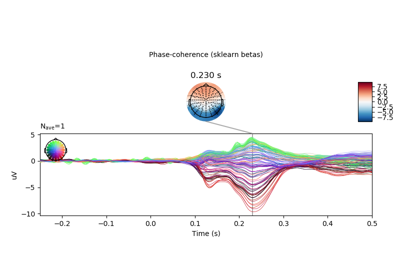Plot bootstrapped confidence interval for linear model fit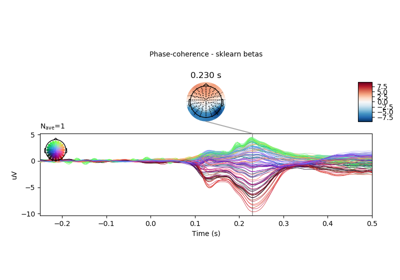Plot beta coefficients from linear model estimation with sklearn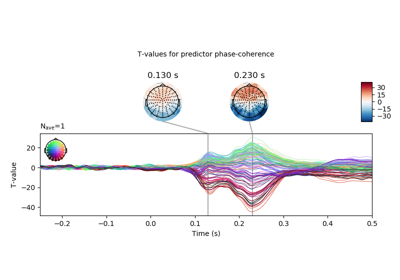Plot p-values for single subject beta coefficients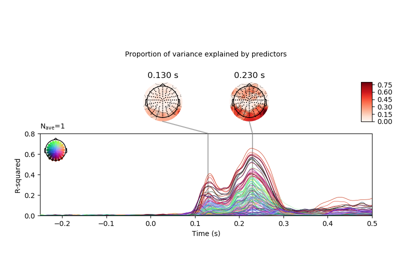Plot R-squared for a linear model

# Group-level analysis¶

This section extends the analysis techniques presented in the single subject analysis section, covering useful tools to estimate linear regression effects on a group level (i.e., including data from multiple subjects).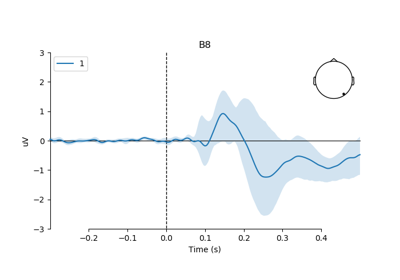Plot group-level effect of continuous covariate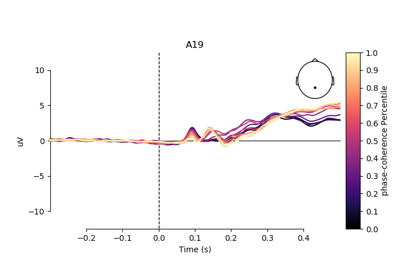Plot group-level ERPs across percentiles of continuous variable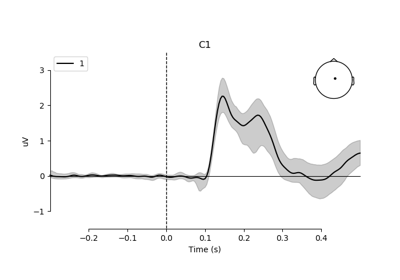Plot group-level effect of continuous variable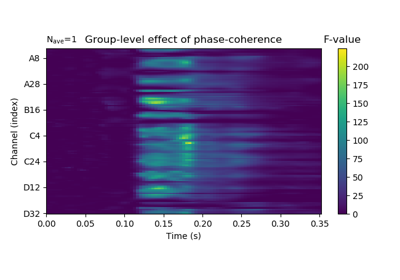Plot spatiotemporal clustering results for effect of continuous variable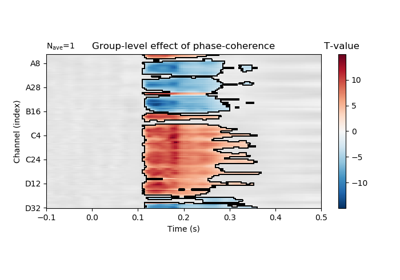Plot significance t-map for effect of continuous variable

Gallery generated by Sphinx-Gallery